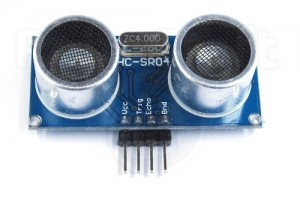Пример:

```// ---------------------------------------------------------------------------
// This example code was used to successfully communicate with 15 ultrasonic sensors. You can adjust
// the number of sensors in your project by changing SONAR_NUM and the number of NewPing objects in the
// "sonar" array. You also need to change the pins for each sensor for the NewPing objects. Each sensor
// is pinged at 33ms intervals. So, one cycle of all sensors takes 495ms (33 * 15 = 495ms). The results
// are sent to the "oneSensorCycle" function which currently just displays the distance data. Your project
// would normally process the sensor results in this function (for example, decide if a robot needs to
// turn and call the turn function). Keep in mind this example is event-driven. Your complete sketch needs
// to be written so there's no "delay" commands and the loop() cycles at faster than a 33ms rate. If other
// processes take longer than 33ms, you'll need to increase PING_INTERVAL so it doesn't get behind.
// ---------------------------------------------------------------------------
#include "NewPing.h"

#define SONAR_NUM     8 // Number or sensors.
#define MAX_DISTANCE 200 // Maximum distance (in cm) to ping.
#define PING_INTERVAL 33 // Milliseconds between sensor pings (29ms is about the min to avoid cross-sensor echo).

unsigned long pingTimer[SONAR_NUM]; // Holds the times when the next ping should happen for each sensor.
unsigned int cm[SONAR_NUM];         // Where the ping distances are stored.
uint8_t currentSensor = 0;          // Keeps track of which sensor is active.

NewPing sonar[SONAR_NUM] = {       //   Sensor object array. Each sensor's trigger pin, echo pin, and max distance to ping.
NewPing(50, 51, MAX_DISTANCE),   // 1
NewPing(52, 53, MAX_DISTANCE),   // 2
NewPing(38, 37, MAX_DISTANCE),   // 3
NewPing(40, 39, MAX_DISTANCE),   // 4
NewPing(33, 34, MAX_DISTANCE),   // 5
NewPing(35, 36, MAX_DISTANCE),   // 6
NewPing(24, 25, MAX_DISTANCE),   // 7
NewPing(26, 27, MAX_DISTANCE),   // 8
};

void setup() {

Serial1.begin(115200);

pingTimer = millis() + 75;           // First ping starts at 75ms, gives time for the Arduino to chill before starting.

for (uint8_t i = 1; i < SONAR_NUM; i++) // Set the starting time for each sensor.
pingTimer[i] = pingTimer[i - 1] + PING_INTERVAL;
}

void loop() {

for (uint8_t i = 0; i < SONAR_NUM; i++) {       // Loop through all the sensors.
if (millis() >= pingTimer[i]) {               // Is it this sensor's time to ping?
pingTimer[i] += PING_INTERVAL * SONAR_NUM;  // Set next time this sensor will be pinged.
if (i == 0 && currentSensor == SONAR_NUM - 1) oneSensorCycle(); // Sensor ping cycle complete, do something with the results.
sonar[currentSensor].timer_stop();          // Make sure previous timer is canceled before starting a new ping (insurance).
currentSensor = i;                          // Sensor being accessed.
cm[currentSensor] = 0;                      // Make distance zero in case there's no ping echo for this sensor.
sonar[currentSensor].ping_timer(echoCheck); // Do the ping (processing continues, interrupt will call echoCheck to look for echo).
}
}
// The rest of your code would go here.
}

void echoCheck() { // If ping received, set the sensor distance to array.
if (sonar[currentSensor].check_timer())
cm[currentSensor] = sonar[currentSensor].ping_result / US_ROUNDTRIP_CM;
}

void oneSensorCycle() { // Sensor ping cycle complete, do something with the results.

for (uint8_t i = 0; i < SONAR_NUM; i++) {
Serial1.print(i);
Serial1.print("=");
Serial1.print(cm[i]);
Serial1.print("cm ");
}

Serial1.println();
}
```